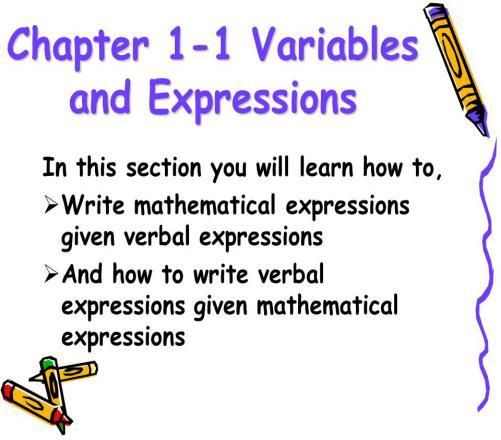# What Do You Know About Variables And Expressions?

Approved & Edited by ProProfs Editorial Team
At ProProfs Quizzes, our dedicated in-house team of experts takes pride in their work. With a sharp eye for detail, they meticulously review each quiz. This ensures that every quiz, taken by over 100 million users, meets our standards of accuracy, clarity, and engagement.
| Written by Jaksiboy
J
Jaksiboy
Community Contributor
Quizzes Created: 924 | Total Attempts: 12,123,294
Questions: 10 | Attempts: 139SettingsVariables and expression are general terms used in mathematics, computer science and many other fields. What do you know about it? How is it used?

• 1.

### Which of the following is a kind of mathematical expression?

• A.

Arithmetic

• B.

Shigelatic

• C.

Neumaic

• D.

None of the above

A. Arithmetic
Explanation
Arithmetic is a kind of mathematical expression that involves numbers and basic operations such as addition, subtraction, multiplication, and division. It is a fundamental branch of mathematics that deals with the manipulation of numbers and their relationships. Arithmetic expressions are commonly used in everyday calculations and mathematical equations.

Rate this question:

• 2.

### Which of the following is known to comprises both numbers and variables together with at least one arithmetic operation?

• A.

Surd

• B.

Algebraic

• C.

All of the above

• D.

None of the above

B. Algebraic
Explanation
Algebraic expressions are known to comprise both numbers and variables together with at least one arithmetic operation. These expressions can involve addition, subtraction, multiplication, division, and exponentiation. Surds, on the other hand, are expressions that involve irrational numbers and do not necessarily contain variables. Therefore, the correct answer is "Algebraic."

Rate this question:

• 3.

### To evaluate an expression means to find its value.

• A.

False

• B.

True

• C.

I don't Know

• D.

Maybe

B. True
Explanation
The given answer is true because evaluating an expression does indeed mean finding its value. When we evaluate an expression, we substitute the variables with their respective values and perform the necessary operations to find the final result. This process allows us to determine the numerical value or outcome of the expression. Therefore, the statement is correct.

Rate this question:

• 4.

### Evaluate 8x – 1 when x = 2.

• A.

1

• B.

7

• C.

8

• D.

15

D. 15
Explanation
To evaluate 8x - 1 when x = 2, we substitute x with 2 in the expression. Therefore, 8(2) - 1 = 16 - 1 = 15.

Rate this question:

• 5.

### Which of the following is known as the study of an expression?

• A.

Syntax

• B.

Semantics

• C.

Express

• D.

None of the abpve

B. Semantics
Explanation
Semantics is the study of the meaning of expressions. It focuses on understanding how words, phrases, and sentences convey meaning and how they relate to the real world. Syntax, on the other hand, is the study of the rules governing the structure and arrangement of words in a language. While syntax is important for understanding the grammatical structure of an expression, semantics is concerned with the interpretation and understanding of its meaning. Therefore, semantics is the correct answer for the study of an expression.

Rate this question:

• 6.

### Evaluate l * w when l = 3 and w = 8

• A.

23

• B.

12

• C.

11

• D.

24

D. 24
Explanation
The given question asks to evaluate the product of l and w when l is 3 and w is 8. To find the product, we simply multiply the two numbers together. In this case, 3 multiplied by 8 equals 24. Therefore, the correct answer is 24.

Rate this question:

• 7.

### Which of the following is defined as a letter that represents unspecified numbers?

• A.

Expression

• B.

Variable

• C.

All of the above

• D.

None of the above

B. Variable
Explanation
A variable is defined as a letter that represents unspecified numbers. In programming or mathematics, variables are used to store and manipulate data. They can take on different values, and their specific values are not known until they are assigned. By using variables, we can write code or equations that can work with different values without having to rewrite the entire code or equation. Therefore, a variable is the correct answer to the question.

Rate this question:

• 8.

### Which of the following is an expression?

• A.

4a+3b

• B.

144

• C.

13+5

• D.

All of the above

A. 4a+3b
Explanation
An expression is a mathematical phrase that contains numbers, variables, and operations. In this case, "4a+3b" is an expression because it consists of variables (a and b) and operations (addition and multiplication). The other options, 144 and 13+5, are not expressions as they are simply numbers or a sum of numbers without any variables or operations. Therefore, the correct answer is 4a+3b.

Rate this question:

• 9.

### In the following equation which of it is variable? 10x +24+3+7=0

• A.

24

• B.

X

• C.

10

• D.

7

B. X
Explanation
The variable in the given equation is represented by "x". It is the unknown quantity that we are trying to solve for in order to make the equation true. The other numbers in the equation, such as 24, 10, and 7, are constants and do not change.

Rate this question:

• 10.

### Evaluate the expression when x=5,  2x2 * 4

• A.

250

• B.

200

• C.

300

• D.

150Back to top